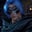Related Tags

communitycreator
javascript

# How to convert negative numbers to positive numbers in JavaScriptGutha Vamsi Krishna

In this shot, we’ll learn how to convert a negative number to a positive number in JavaScript.

We’ll start with the problem statement and then look at an example.

### Problem

Given a negative integer n, convert it to a positive integer.

#### Example

Input: -20

Output: 20

### Solution

We will solve this problem in two ways:

1. Multiply the number with -1.
2. Use the abs() method.

### Multiply with -1

In this approach, we’ll convert a negative integer to a positive integer by multiplying the negative integer with -1.

#### Implementation

//given negative integer
let n = -20;

//print original n
console.log("Given negative integer n = " + n);

//convert n to positive integer
n = n * -1

//print positive n
console.log("After conversion value of n  = " + n)

#### Explanation

Let’s look at the explanation of the code snippet above.

• Line 2: We set the value of the integer n as -20.

• Line 5: We print the original integer n.

• Line 8: We multiply the negative integer by -1 and assign the result to n.

• Line 11: We print the converted positive integer n.

### Use the abs() method

In this approach, we’ll use a built-in method provided by the Math module in JavaScript.

The method Math.abs() converts the provided negative integer to a positive integer.

#### Implementation

//given negative integer
let n = -20;

//print original n
console.log("Given negative integer n = " + n);

//convert n to positive integer
n = Math.abs(n)

//print positive n
console.log("After conversion value of n  = " + n)

#### Explanation

Let’s look at the explanation of the code snippet above.

• Line 2: We set the value of the integer n as -20.

• Line 5: We print the original integer n.

• Line 8: We pass n as an argument to the Math.abs() method and assign the result to n.

• Line 11: We print the converted positive integer n.

RELATED TAGS

communitycreator
javascript

CONTRIBUTORGutha Vamsi Krishna
RELATED COURSES

View all Courses

Keep Exploring

Learn in-demand tech skills in half the time